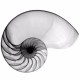# A Skeleton Multiplication Puzzle by Knuth

I want to share with you two ways to solve an interesting puzzle from Don Knuth’s “The Art of Computer Programming“. Here is the first!

The puzzle that caught my attention, quite by chance, is the “skeleton multiplication puzzle” given in Exercise 50 of Pre-Fascicle 9b (called “A Potpourri of Puzzles”). We want to find two numbers whose product is 777777, but we are given very little information. Here is the puzzle:

```        N N N  (1)
x N N N N  (2)
---------
N N N 7  (3)
N N N N    (4)
N N N N      (5)
N N N        (6)
-----------
7 7 7 7 7 7  (N != 7)```

All of the “Ns” are unknown, and none of them can be a 7. Notice that I’ve numbered the rows (1) – (6) because I am going to obsess about them below.

This doesn’t seem like a lot to go on, does it? I’ll give you some space to think about it, then I will show you Knuth’s solution.

….

OK, here’s what Knuth says:

“The only 3-digit divisors of 777777 = 3 x 7 x 7 x 11 x 13 x 37 that contain no 7s and don’t end in 1 are (143, 259, 429, 539). Their cofactors are (5439, 3003, 1813, 1443). And only 539 x 1443 gives just seven 7s.”

So that settles that, right? Well, not quite. Let’s dig into the details.

Let’s call the number in row (1) n_1, and the number in row (2) n_2. We want n_1 * n_2 = 777777.

Remember (or perhaps learn for the very first time…) that for every positive integer, there is a unique set of primes whose product is that number. This is called the prime factorization of a number.  We can use the prime factorization of 777777 to narrow down the possibilities for n_1 and n_2.

From Wolfram Alpha, we can get the prime factors: (3, 7, 7, 11, 13, 37).

Since n_1 * n_2 = 777777,  the prime factors of n_1 and n_2 also come from this set. Let’s focus on n_1. If I go through all the subsets of (3, 7, 7, 11, 13, 37) whose product has three digits, here’s what I find. Hopefully I didn’t miss any!

7 * 37 = 259
11 * 13 = 143
11 * 37 = 407
13 * 37 = 481
3 * 7 * 7 = 147
3 * 7 * 11 = 231
3 * 7 * 13 = 273
3 * 11 * 13 = 429
11 * 13 = 143
3 * 7 * 37 = 777
7 * 7 * 11 = 539
7 * 7 * 13 = 637

But remember that N != 7, so that means:

• n_1 can’t have a 7 as a digit
• The last number of n_1 cannot be 1, otherwise the last digit of n_2 would be 7, because the last digit of the product is 7: look at row (3).

So now we’re down to these possibilities:

259 (cofactor is 777777/259=3003)
143 (cofactor 5439)
429 (cofactor 1813)
539 (cofactor 1443)

But a full solution has to avoid 7s in rows (3)-(6). So we need to work out the long multiplication to see which of these possibilities are actually solutions. Go ahead and do that on paper now, or you can cheat here. When you do, you will see that Knuth’s solution using 539 and 1443 does solve the puzzle. Of course it does; he’s Don Frigging Knuth.

```        4 2 9  (1)
x 1 8 1 3  (2)
---------
1 2 8 7  (3)
0 4 2 9    (4)
3 4 3 2      (5)
4 2 9        (6)
-----------
7 7 7 7 7 7  ```

Hey, this works too! This new solution is a bit strange because it has a leading zero in row (4), but nobody said I can’t do that. When I wrote Prof. Knuth, he agreed.

There is one more plot twist. It turns out that what I have written here is *not* the way I discovered this alternate solution; I am far too lazy for that. Instead, I used Python to do the thinking for me. Read more next time!